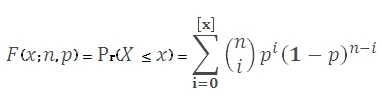# SQL Server BINOM.DIST function

Updated: 9 August 2010

Use BINOMDIST to calculate the binomial distribution. The binomial distribution is the discrete probability distribution of the number of successes in a sequence of n independent yes/no experiments, each of which yields success with probability p. The formula for the probability density function is:The cumulative distribution function is:Syntax
SELECT [wctStatistics].[wct].[BINOMDIST] (
<@Number_s, float,>
,<@Trials, float,>
,<@Probability_s, float,>
,<@Cumulative, bit,>)
Arguments
@Number_s
is the number of successes in trials. @Number_s is an expression of type float or of a type that can be implicitly converted to float
@Trials
is the number of independent trials. @Trials is an expression of type float or of a type that can be implicitly converted to float
@Probability_s
is the probability of success in each trail. @Probability_s is an expression of type float or of a type that can be implicitly converted to float
@Cumulative
is a logical value that determines the probability density function (False, 0) or the cumulative distribution function (True, 1) is being calculated.
Return Types
float
Remarks
·         @Number_s and @Trials are truncated to zero decimal places.
·         If @Number_s, @Trials, or @Probability_s <0, BINOMDIST returns an error.
·         If @Number_s > @Trials, BINOMDIST returns an error.
·         If @Probability_s > 1, BINOMDIST returns an error.
·         If @Cumulative = False then BINOMDIST =
BICO(@Trials,@trials-@number_s)*POWER(@Probability_s,@Number_s)*POWER((1-@Probability_s),(@Trials-@Number_s))
·         If @Cumulative = True then BINOMDIST =
Examples

select
wct.BINOMDIST(6,10,.5,'False')

This produces the following result

----------------------
0.205078125000001

(1 row(s) affected)

select wct.BINOMDIST(6,10,.5,'True')

This produces the following result

----------------------
0.828125

(1 row(s) affected)

select wct.BICO(10, 10-6)
*POWER(.500000000,6.00000000)
*POWER(1.00000000-.500000000,10.0000000-6.0000000)

This produces the following result
----------------------
0.205078125

(1 row(s) affected)

select 1 - wct.betadist(.5,6+1, 10 -6, null,null)

This produces the following result

----------------------
0.828124999999999

(1 row(s) affected)

### SupportCopyright 2008-2021 Westclintech LLC         Privacy Policy        Terms of Service